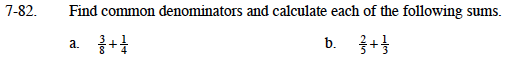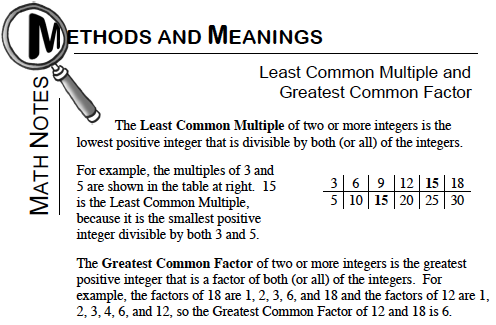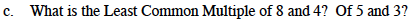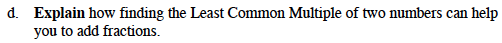### Home > MC1 > Chapter 7 > Lesson 7.2.4 > Problem7-82

7-82.Remember, the denominator is the bottom number of a fraction. Here the denominators are 8 and 4.
Can you change the fractions so that both denominators are 8?

$\text{The answer to part (a) is }\frac{5}{8}.$

Here, you might like to find the Least Common Multiple of 5 and 3. This number should eventually be your common denominator. Refer to the Math Notes box below.$\text{The common denominator is 15. Knowing that there are }\frac{3}{15}\text{'s in }\frac{1}{5}\text{ and }\frac{5}{15}\text{ in }\frac{1}{3},$

$\text{can you change the fractions to be equal to }\frac{\textit{x}}{15}?\text{ Use multiplication and the given numerators.}$

$\text{If }\frac{2}{5}+\frac{1}{3}=\frac{6}{15}, \text{ can you find the final sum?}$Least Common Multiples are often also common denominators. Refer to parts (a),(b), and the Math Notes box can you answer this question?Look back at parts (a), (b), and (c). How have Least Common Multiples come in handy? Noticing this pattern can help you to explain your answer here.